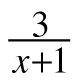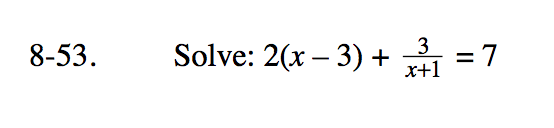### Home > PC3 > Chapter 8 > Lesson 8.1.3 > Problem8-53

8-53.

Solve: 2(x – 3) += 7 Homework Help ✎Multiply each term by (x + 1).

Once you expand the terms and simplify, you will need to use the Quadratic Formula to solve the equation.